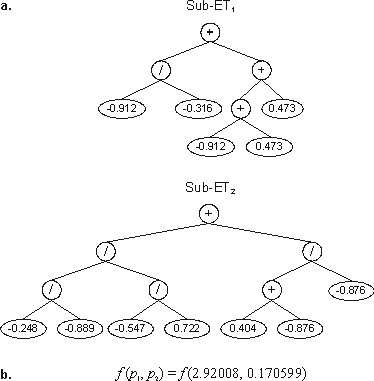Home
News
Author
Q&A
Tutorials
GEP Biblio
Contacts

Visit Gepsoft

Gene Expression Programming: Mathematical Modeling by an Artificial Intelligence

Multigenic chromosomes and multidimensional parameter optimization

The multigenic structure of gene expression programming can be fruitfully exploited to solve function optimization problems. In this case, the values of the N parameters of a function are encoded in N different genes.

Genetic algorithms have been widely used for parameter optimization (see, e.g., Goldberg 1989 and Haupt and Haupt 1998). In that case, the simple chromosomes of GAs encode the values of the different parameters. Whether in binary or floating-point format, the different parameters of a function are directly encoded in the simple GA chromosomes. In GEP, however, the best parameters are found through mathematical operations performed on an ever-changing set of random numerical constants. This approach offers, in my view, new possibilities as one can exploit the vast set of GEP genetic operators to fine-tune the different parameters and discover the appropriate range for the constants.

Thus, to solve this kind of problem using GEP, we are going to use the chromosomal organization introduced in the previous section for handling random numerical constants. For most optimization problems, the set of functions F required is usually very simple and consists of the basic arithmetic operators. The set of terminals consists only of the ephemeral random constant and thus T = {?}. The set of random numerical constants is also easy to choose and, usually, a set of 10 random constants works very well for most problems, giving R = {0, 1, 2, 3, 4, 5, 6, 7, 8, 9}. And, finally, the ephemeral random constant “?” ranges usually over the interval [-1, 1]. This set of parameters is almost a universal receipt for function optimization problems using GEP, for the algorithm itself is used to discover the appropriate range for the constants.

Consider the chromosome below, composed of two genes:

 Gene 0: +/+??+???????4374796         A0 = {-0.698, 0.781, -0.059, -0.316, -0.912, 0.398, 0.157, 0.473, 0.103, -0.756}     Gene 2: +////+???????4562174 A2 = {0.104, 0.722, -0.547, -0.052, -0.876, -0.248, -0.889, 0.404, 0.981, -0.149} (4.19)

Its expression is shown in Figure 4.9. As you can see, the first gene encodes the value 2.92008 for the first parameter p1, and the second encodes the value 0.170599 for the second parameter p2.Figure 4.9. Expression of chromosomes encoding parameter values in a function optimization task. a) The sub-ETs encoded in program (4.19). b) The values of the different parameters encoded in each gene. The fitness of this program is the function value at point (p1, p2).

The fitness of this kind of chromosome consists of f(p1, p2), where f is the function we are trying to optimize. Depending on the function, the value returned for a particular set of parameters can be anywhere in the real line and, therefore, something must be done about negative or zero fitnesses. To solve this problem, for each generation, the worse-of-generation fitness fmin is evaluated and, if fmin is negative, the absolute value of fmin plus 1 is added to the fitness. This way one guarantees that all the individuals will have positive fitness, with the less fit having fitness equal to one. This transformed fitness can now be used to select individuals to reproduce with modification.

Home | Contents | Previous | Next